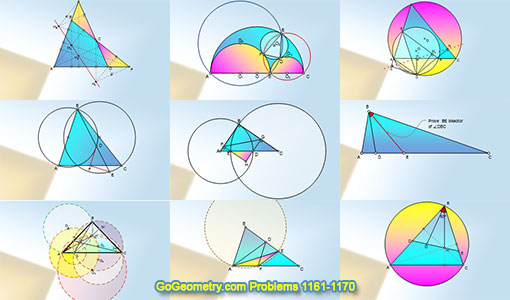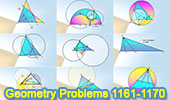# Geometry Problems 1161-1170. Level: High School, CollegeGeometry Problems 1161 - 1170Geometry Problem 1161. Scalene Triangle, 60 Degrees, Angle, Orthocenter, Circumcenter, Equilateral Triangle, Congruence. Geometry Problem 1162. Triangle, Congruence, Double Angle, Parallel Lines, Circle, Circumcenter. Geometry Problem 1163. Isosceles Triangle, Congruence, Double Angle, Circle, Concyclic Points, Circumcenter, Perpendicular, Angle Bisector. Geometry Problem 1164. Triangle, Angle Bisector, Cevian. Typography of Geometry Problem 1164 using iPad Apps. Geometry Problem 1165. Right Triangle, Circle, Center, Radius, Cathetus, Perpendicular, 90 Degrees, Perimeter, Metric Relations. Typography of Geometry Problem 1165 using iPad Apps. Geometry Problem 1166. Triangle, Circles, Diameters, Secant, Midpoint, Congruence. Geometry Problem 1167. Triangle, Circle, Circumcircle, Perpendicular, 90 Degrees, Collinear Points. Geometry Problem 1168 Construction of the Inscribed Circle of the Arbelos, Semicircles, Diameter, Circle, Triangle, Circumcircle, Tangent. Geometry Problem 1169 Complete Quadrilateral, Orthocenters of the Component Triangles, Collinear Points, Ortholine, Steiner-line, Orthocentric line. Geometry Problem 1170 Area of a Triangle in terms of the three sides, a-b-c.

Home | Sitemap | Search | Geometry | Problems | Visual Index | 10 ProblemsEmail | Post a comment | by Antonio Gutierrez
Last updated: Nov 19, 2017